# Modeling and Control of a Mars Rover

This example models a mars rover performing a sample retrieval task using Simscape Multibody and Robotics System Toolbox. The rover follows a desired path on a rigid terrain surface, stops at the target location and uses its manipulator to pick and store a sample from the surface. It uses the following key features to model different aspects of the application:

### Mars Rover Model

Refer to the model `sm_mars_rover.slx` to view the subsystems mentioned in this example.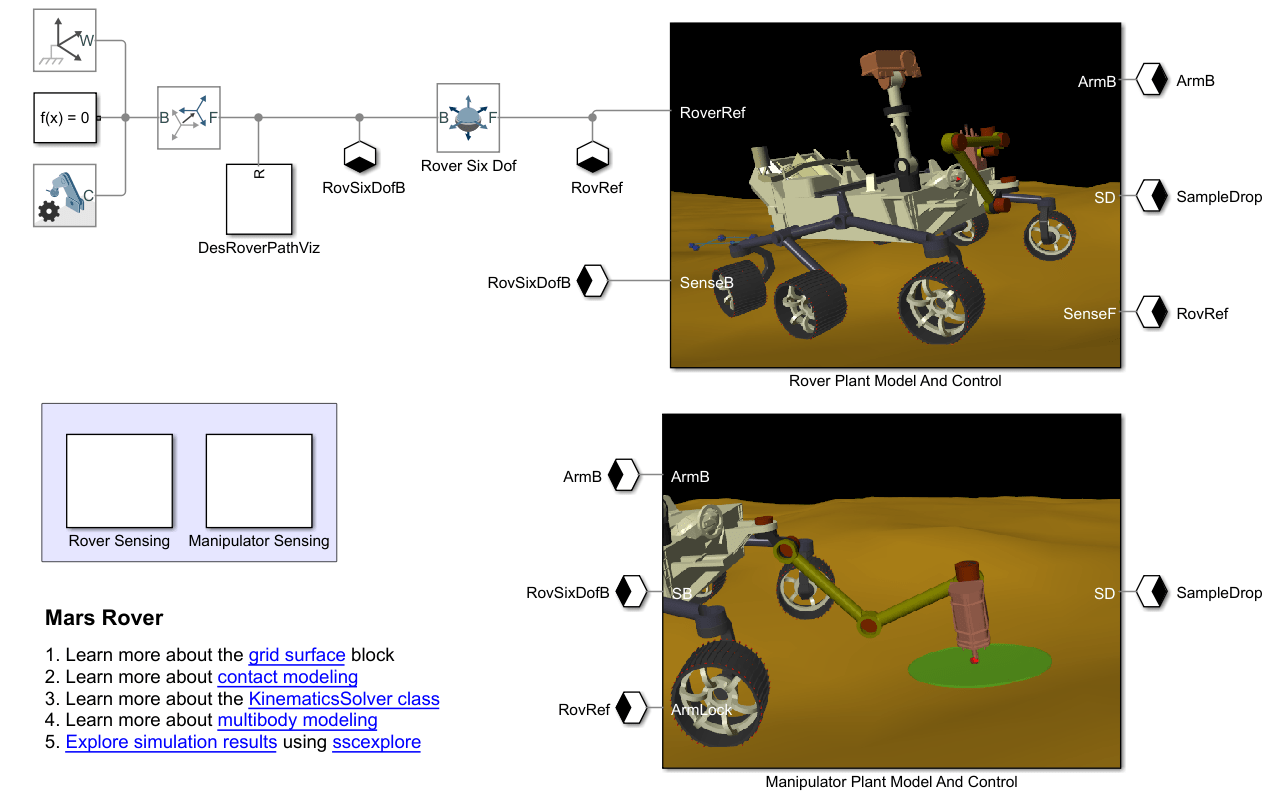### Mars Rover Animation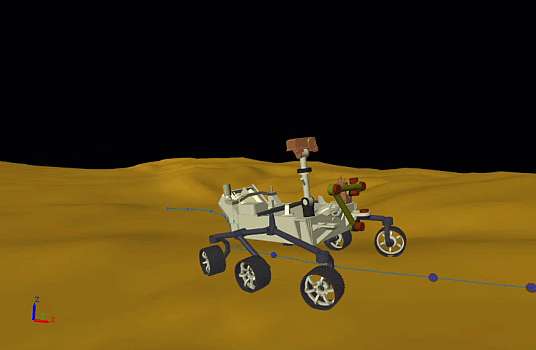### Rover Plant Model and Control

This subsystem models the rover, rigid terrain surface and the path planning and controls aspects of the system.#### Rover Subsystem

This subsystem models various components of the rover like chassis, rocker-bogie suspension and wheels. The CAD parts for the geometry are imported into Simscape Multibody using the File Solid.

The rover's actuators correspond to the six torque-actuated revolute joints mounted to each of the six wheels for speed control and the four torque-actuated revolute joints mounted to the top of four corner wheels used for steering. In addition to this, three main components of the suspension mechanism are also modeled, namely the differential arm, rocker and bogie.

The contact between the wheels and the rigid terrain is modeled using Point Cloud and Grid Surface contact pairs along with the Spatial Contact Force block. The points on each wheel's grousers are created using the Point Cloud block.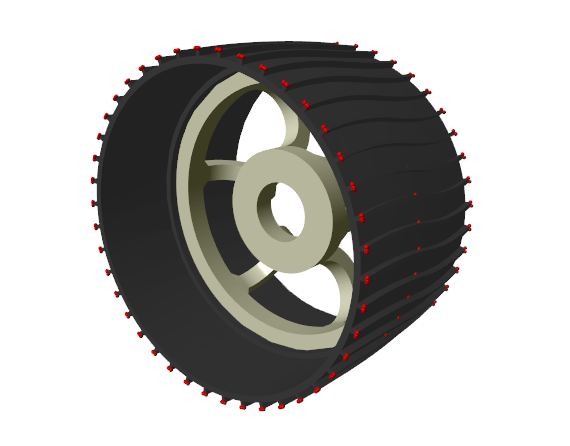#### Rigid Terrain Surface

To model a Martian surface, a rigid terrain is created using the Grid Surface block. Refer to the file `rover_rigid_terrain_params.m` to setup the parameters needed to create the Grid Surface from a STL file.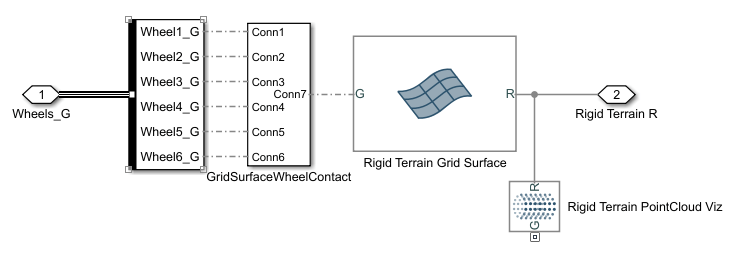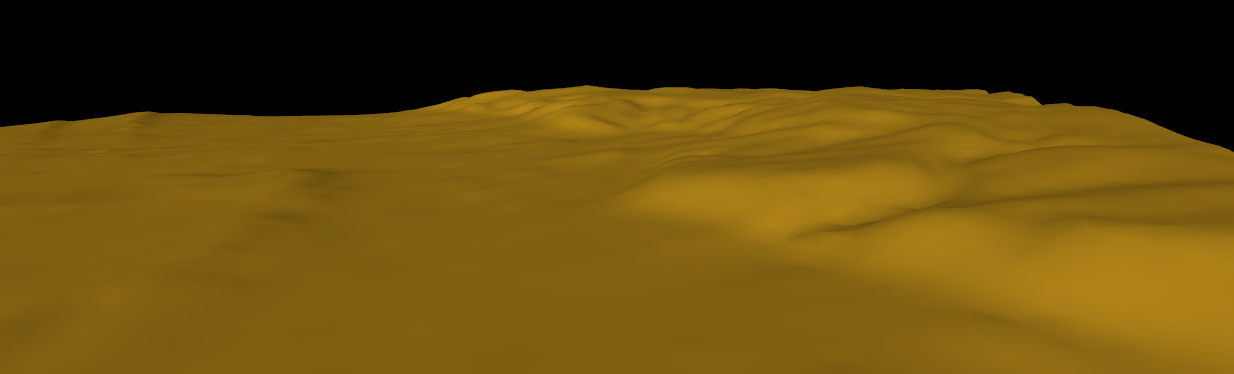#### Rover Path Planning and Control

This subsystem models rover's path tracking control system. The path consists of ordered waypoints in the X-Y plane which the rover is desired to pass through. These waypoints are assumed to be provided by a high-level path planner and would represent an obstacle free path for the rover. These waypoints can be loaded using the `roverDesiredPath.mat` file.

The goal of this subsystem is to first, compute the necessary steering angles and the wheel speeds needed to follow a desired path and a desired chassis linear velocity and second, to compute the necessary actuator torques needed to achieve these steering angles and wheel speeds.

For developing the path tracking controller, the following considerations are made:

• Mars rovers are typically assumed to have low forward velocity (on the order of cm/s), therefore the dynamics of the motion are ignored and the controls problem is approached using kinematic equations only.

• To simplify the kinematics formulation, the rover is assumed to be moving on a planar surface.

• The four corner wheels of the rover have independent steering which can enable the rover to perform Ackerman steers. Based on this capability, the rover is considered to be using Ackerman steering.

• The Ackerman steering geometry is simplified by assuming a 2D geometric bicycle model with an equivalent turn radius. This simplification is done by representing each pair of wheels by a single wheel located in the middle and a single steering angle corresponding to the turn radius of the center of the rover.

• The front and the rear wheels are considered to be steered symmetrically.

• The wheels are assumed to roll without slipping.

Based on the above considerations, a six wheel rover can be equivalently represented by a geometric bicycle model.

Pure Pursuit (Robotics System Toolbox) is used for path tracking. This is a geometric algorithm that computes a target direction angle ($\alpha$) needed to move the robot from its current position to reach some look-ahead point in front of the robot.

#### Steering Angles Formulation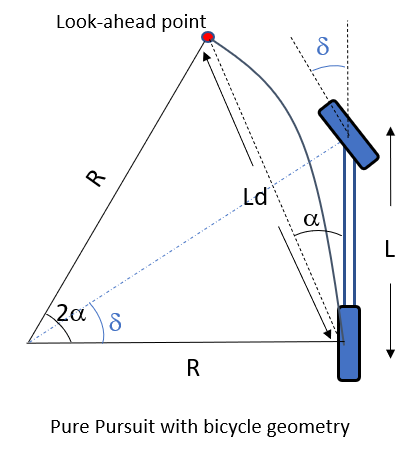The steering angles for each of the four corner wheels of the rover are derived in two steps. We first use the geometric bicycle model and the target direction angle ($\alpha$) (provided by the Pure Pursuit Controller) to obtain the bicycle steering angle ($\delta$) and the turn radius ($\mathit{R}$) as shown below.

`$\delta \text{\hspace{0.17em}}={\mathrm{tan}}^{-1}\left(\frac{2\mathit{L}\text{\hspace{0.17em}}\mathrm{sin}\left(\alpha \right)}{{\mathit{L}}_{\mathit{d}}}\right)$`

`$\mathit{R}\text{\hspace{0.17em}}=\frac{\mathit{L}}{\mathrm{tan}\left(\delta \right)}$`

where,

`$\begin{array}{l}\alpha :\mathrm{Target}\text{\hspace{0.17em}}\mathrm{Dir}\text{\hspace{0.17em}}\mathrm{Angle}\\ \delta :\mathrm{Bicycle}\text{\hspace{0.17em}}\mathrm{Steering}\text{\hspace{0.17em}}\mathrm{Angle}\\ \mathit{L}:\mathrm{Bicycle}\text{\hspace{0.17em}}\mathrm{Length}\\ {\mathit{L}}_{\mathit{d}}:\mathrm{Look}\text{\hspace{0.17em}}\mathrm{ahead}\text{\hspace{0.17em}}\mathrm{distance}\text{\hspace{0.17em}}\mathrm{for}\text{\hspace{0.17em}}\mathrm{Pure}\text{\hspace{0.17em}}\mathrm{Pursuit}\\ \mathit{R}:\mathrm{Turn}\text{\hspace{0.17em}}\mathrm{radius}\text{\hspace{0.17em}}\mathrm{of}\text{\hspace{0.17em}}\mathrm{rover}\text{\hspace{0.17em}}\mathrm{center}\end{array}$`Based on the computed bicycle steering angle ($\delta$) and the turn radius of the rover($\mathit{R}$), we then obtain the individual steering angles (${\delta }_{\mathrm{LF}}\text{\hspace{0.17em}},{\delta }_{\mathrm{LB}}\text{\hspace{0.17em}},{\delta }_{\mathrm{RF}},{\delta }_{\mathrm{LB}}$) using the Ackerman steering geometry.

`$\text{\hspace{0.17em}}{\delta }_{\mathrm{LF}}\text{\hspace{0.17em}}={\mathrm{tan}}^{-1}\left(\frac{\mathit{L}}{\mathit{R}-\mathit{d}}\right)$`

`${\text{\hspace{0.17em}}\delta }_{\mathrm{RF}}\text{\hspace{0.17em}}={\mathrm{tan}}^{-1}\left(\frac{\mathit{L}}{\mathit{R}+\mathit{d}}\right)$`

${\text{\hspace{0.17em}}\delta }_{\mathrm{LB}}$ = -${\delta }_{\mathrm{LF}}$

${\text{\hspace{0.17em}}\delta }_{\mathrm{RB}}$ = -${\delta }_{\mathrm{RF}}$

where,

`$\begin{array}{l}{\delta }_{\mathrm{LF}}\text{\hspace{0.17em}},{\delta }_{\mathrm{LB}}\text{\hspace{0.17em}},{\delta }_{\mathrm{RF}},{\delta }_{\mathrm{LB}}:\mathrm{Steering}\text{\hspace{0.17em}}\mathrm{angles}\text{\hspace{0.17em}}\mathrm{of}\text{\hspace{0.17em}}\mathrm{respective}\text{\hspace{0.17em}}\mathrm{corner}\text{\hspace{0.17em}}\mathrm{wheels}\\ \mathit{L}:\mathrm{Bicycle}\text{\hspace{0.17em}}\mathrm{Length}\text{\hspace{0.17em}}\left(0.5*\mathrm{Chassis}\text{\hspace{0.17em}}\mathrm{Length}\right)\\ \mathit{d}:0.5*\mathrm{Chassis}\text{\hspace{0.17em}}\mathrm{Width}\\ \mathrm{ICR}:\mathrm{Instantenous}\text{\hspace{0.17em}}\mathrm{Center}\text{\hspace{0.17em}}\mathrm{of}\text{\hspace{0.17em}}\mathrm{Rotation}\text{\hspace{0.17em}}\end{array}$`

#### Wheel Speed Formulation

Based on the Ackerman steering geometry and the turn radius ($\mathit{R}$), we also obtain the relationship between the chassis speed ($\mathrm{Vc}$) and the wheel speeds ($\omega$) as shown below  :

`${\omega }_{\mathrm{LF}\text{\hspace{0.17em}}}=\frac{{\mathit{V}}_{\mathit{C}}}{{\mathit{R}}_{\mathit{w}}}*\frac{\left(\sqrt{{\mathit{L}}^{2}+{\left(\mathit{R}-\mathit{d}\right)}^{2}}\right)}{\mathit{R}}\text{\hspace{0.17em}\hspace{0.17em}}$`

`${\omega }_{\mathrm{RF}\text{\hspace{0.17em}}}=\frac{{\mathit{V}}_{\mathit{C}}}{{\mathit{R}}_{\mathit{w}}}*\frac{\left(\sqrt{{\mathit{L}}^{2}+{\left(\mathit{R}+\mathit{d}\right)}^{2}}\right)}{\mathit{R}}\text{\hspace{0.17em}\hspace{0.17em}}$`

`${\omega }_{\mathrm{LM}\text{\hspace{0.17em}}}=\frac{{\mathit{V}}_{\mathit{C}}}{{\mathit{R}}_{\mathit{w}}}*\frac{\left(\mathit{R}-\mathit{d}\right)}{\mathit{R}}\text{\hspace{0.17em}\hspace{0.17em}}$`

`${\omega }_{\mathrm{RM}\text{\hspace{0.17em}}}=\frac{{\mathit{V}}_{\mathit{C}}}{{\mathit{R}}_{\mathit{w}}}*\frac{\left(\mathit{R}+\mathit{d}\right)}{\mathit{R}}\text{\hspace{0.17em}\hspace{0.17em}}$`

`${\omega }_{\mathrm{LB}\text{\hspace{0.17em}}}={\omega }_{\mathrm{LF}\text{\hspace{0.17em}}}\text{\hspace{0.17em}\hspace{0.17em}}$`

`${\omega }_{\mathrm{RB}\text{\hspace{0.17em}}}={\omega }_{\mathrm{RF}\text{\hspace{0.17em}}}$`

where,

`$\begin{array}{l}\text{\hspace{0.17em}}{{\omega }_{\mathrm{LF}},{\omega }_{\mathrm{LM}},{\omega }_{\mathrm{LB}},{\omega }_{\mathrm{RF}},{\omega }_{\mathrm{RM}},{\omega }_{\mathrm{RB}}}_{\text{\hspace{0.17em}}}:\mathrm{Angular}\text{\hspace{0.17em}}\mathrm{speeds}\text{\hspace{0.17em}}\mathrm{of}\text{\hspace{0.17em}}\mathrm{respective}\text{\hspace{0.17em}}\mathrm{wheels}\text{\hspace{0.17em}}\\ \mathrm{Vc}:\mathrm{Linear}\text{\hspace{0.17em}}\mathrm{speed}\text{\hspace{0.17em}}\mathrm{of}\text{\hspace{0.17em}}\text{\hspace{0.17em}}\mathrm{the}\text{\hspace{0.17em}}\mathrm{rover}\text{\hspace{0.17em}}\mathrm{chassis}\\ {\mathit{R}}_{\mathit{w}}:\mathrm{Radius}\text{\hspace{0.17em}}\mathrm{of}\text{\hspace{0.17em}}\mathrm{the}\text{\hspace{0.17em}}\mathrm{wheel}\end{array}$`

Once both the steering angles and the wheel speeds are formulated for the desired path and the desired chassis linear velocity, a PID controller is used to drive the actual steering angles and the angular rates of the actuators (revolute joints) to their desired values.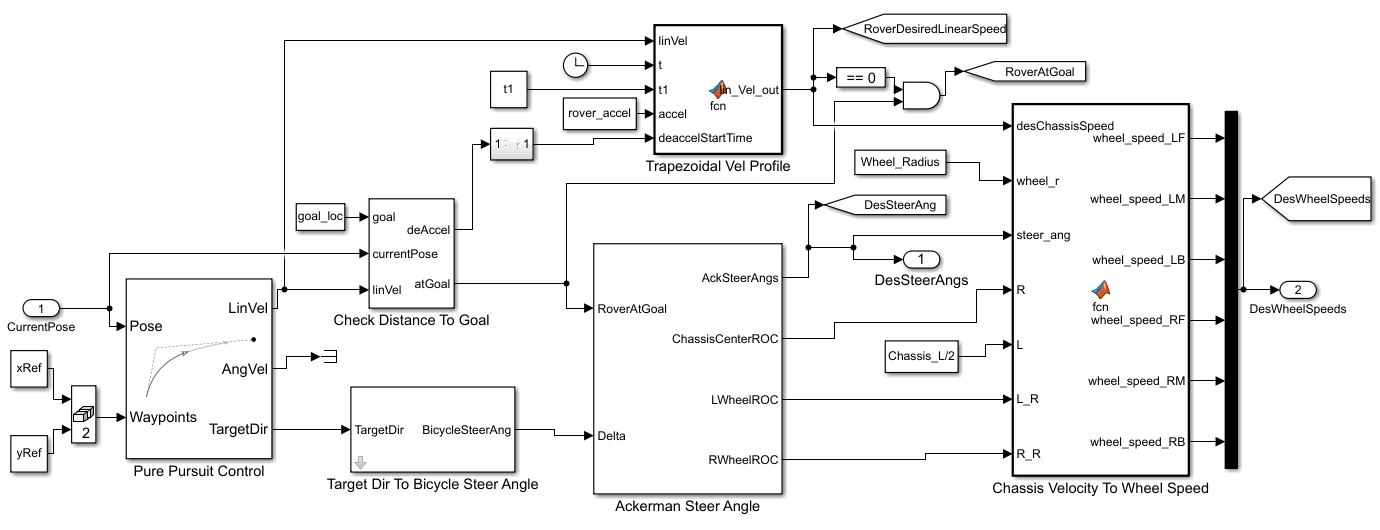#### Rover Simulation Results: Path 1

Results for path 1 when the rover is moving at ~0.3 m/s on an uneven terrain. More quantities can be viewed at Rover Sensing subsystem in the model.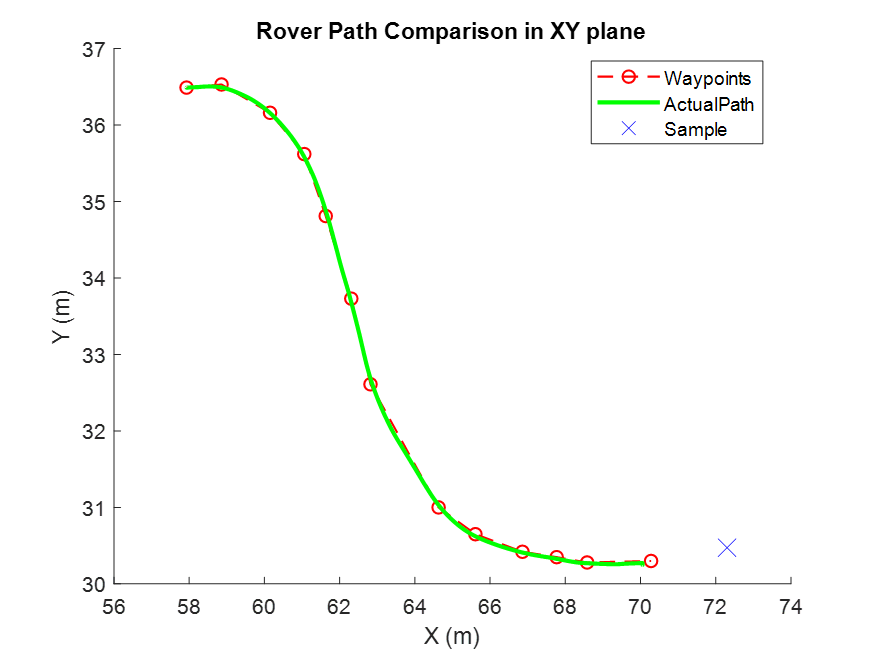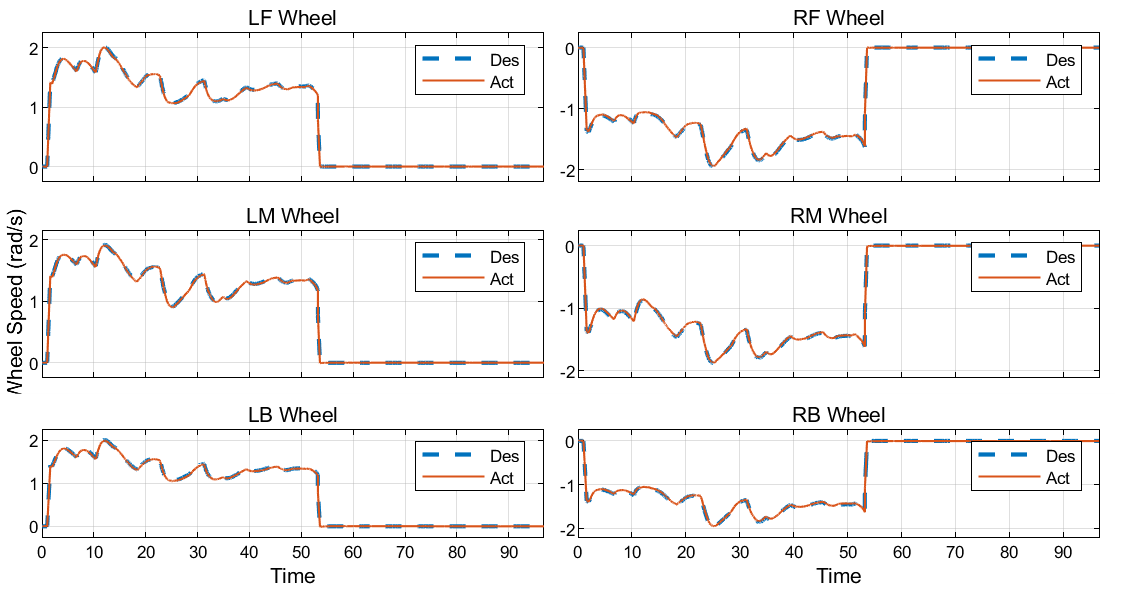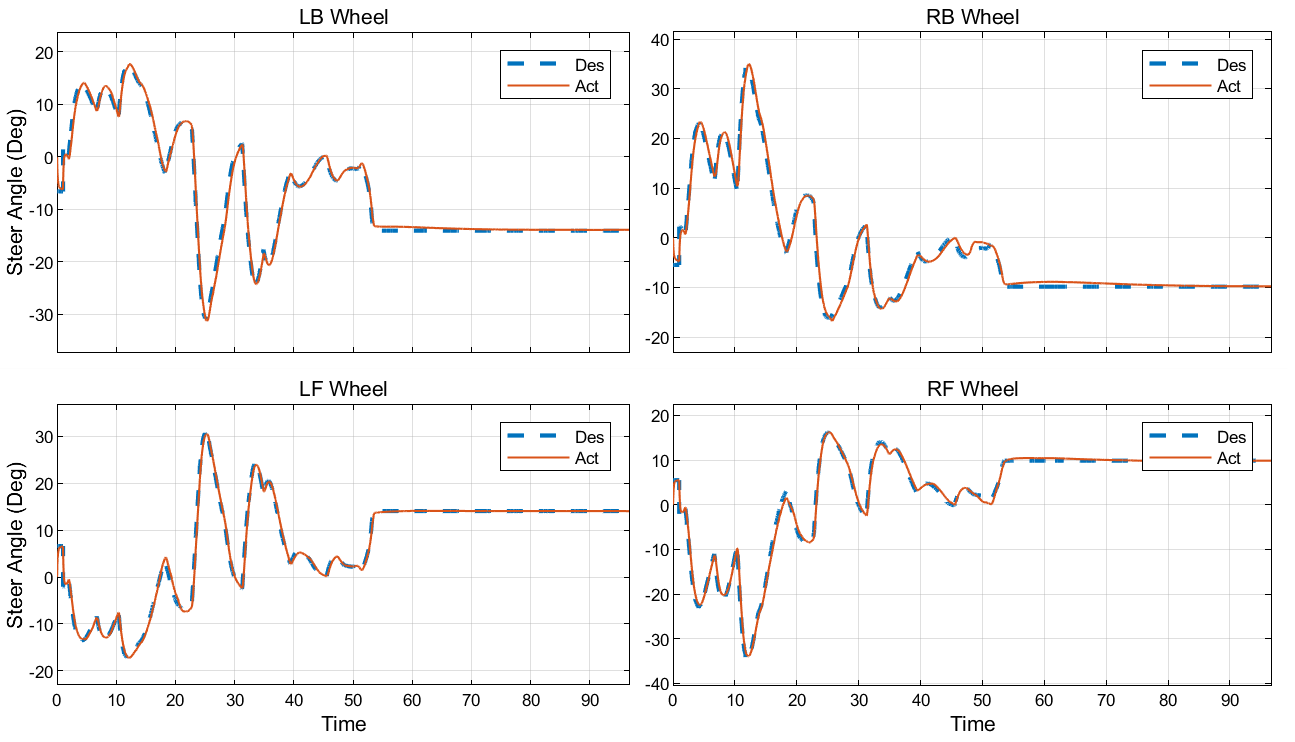#### Rover Simulation Results: Path 2

Results for path 2 when the rover is moving at ~0.3 m/s on an uneven terrain. More quantities can be viewed at Rover Sensing subsystem in the model.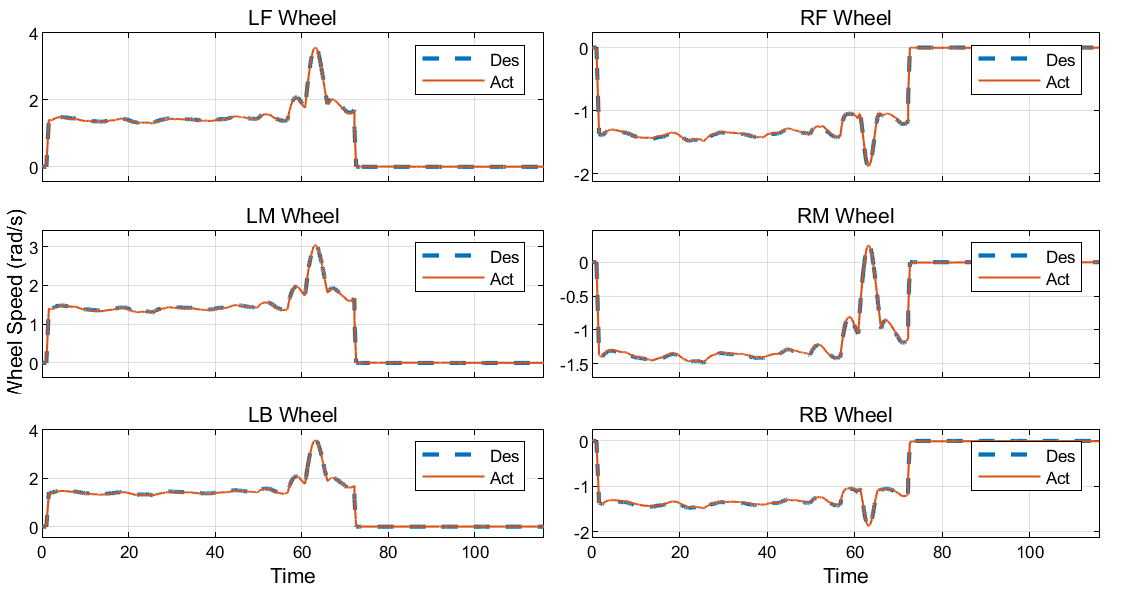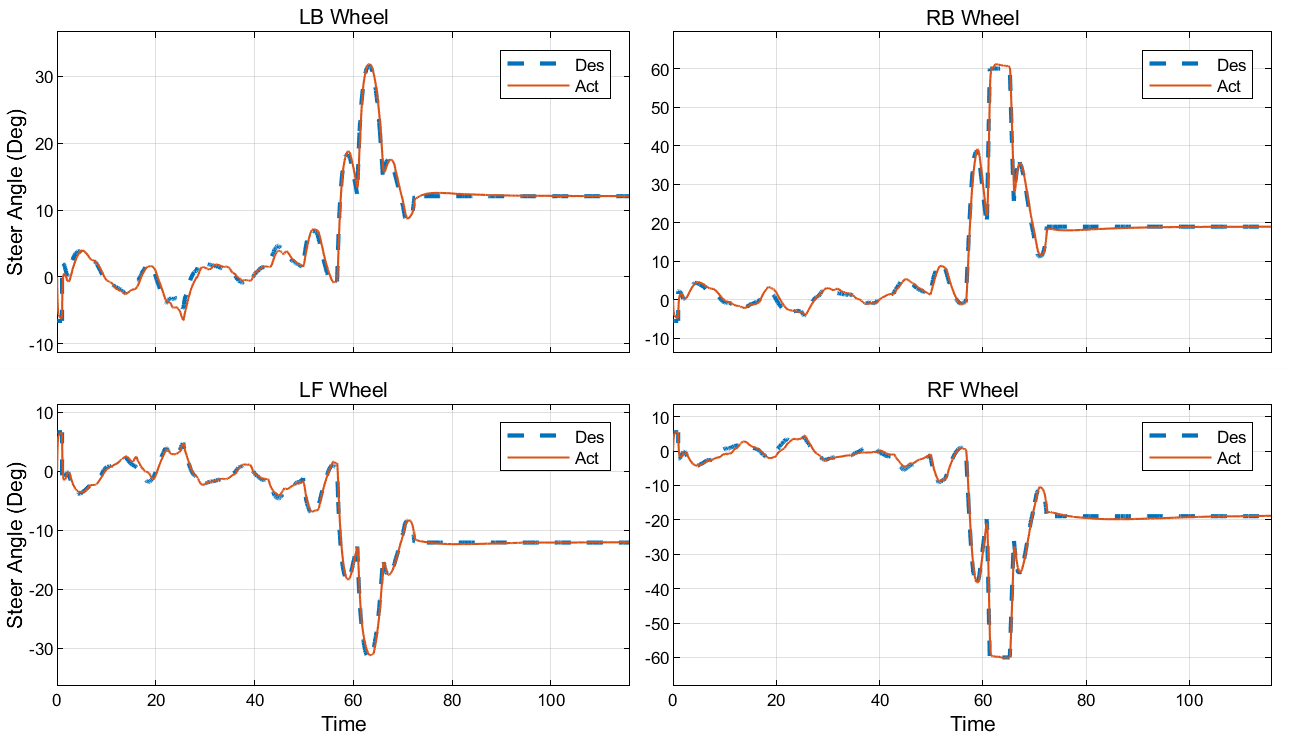### Manipulator Plant Model and Control

This subsystem models the rover's robot arm and its trajectory planning and control to pick and collect a sample from the surface.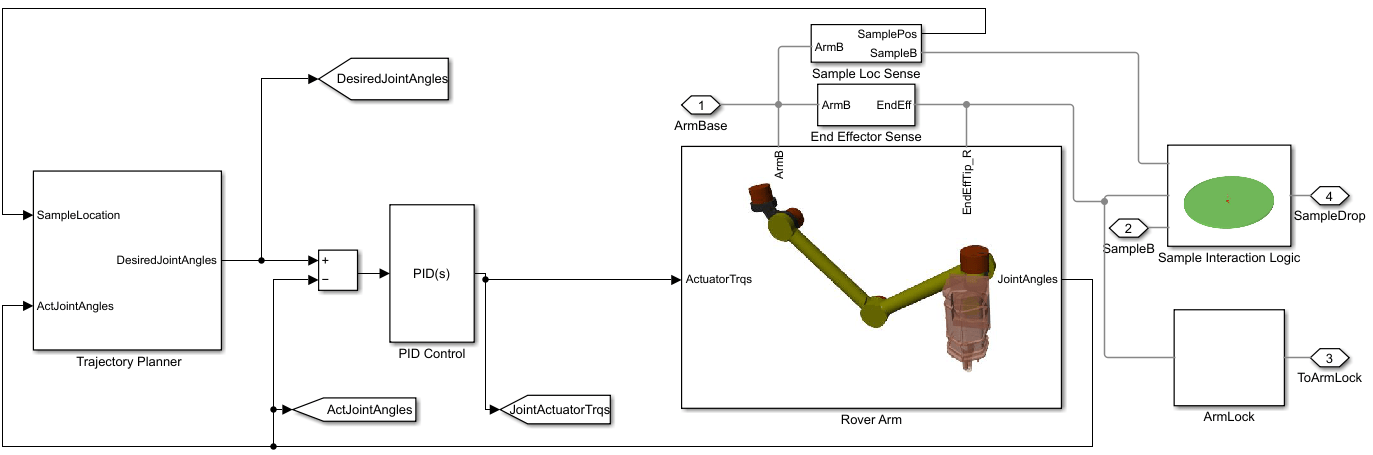#### Rover Arm

The manipulator is modeled as a 6-DOF arm mounted on the front end of the chassis. Its actuators correspond to six torque-actuated revolute joints. To mimic sensors like encoders, the subsystem outputs the joint angles from each of the six revolute joints. We use a simplified model for the interaction between the end effector and the sample that leverages joint mode switching. When the end effector is sufficiently close to the sample, an initially disengaged 6-DOF joint connecting them becomes engaged. This 6-DOF joint has tight position limits to keep the sample nearly constrained to the end effector. When the end effector containing the sample comes sufficiently close to the sample storage location, the engaged 6-DOF joint between the end effector and the sample is disengaged and an initially disengaged 6-DOF joint between the sample and the storage location is engaged. This 6-DOF joint also has tight position limits to keep the sample nearly constrained to the storage location on the chassis.

#### Planning and Control

The manipulator planning and control subsystems are enabled once the rover stops at the target position. Once the rover stops, the sample's location with respect to the arm base is computed using a Transform Sensor block (to mimic the camera on board).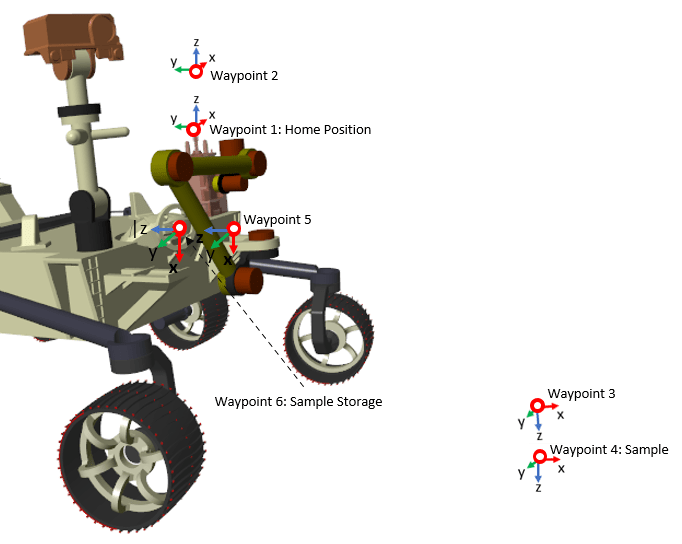To plan the trajectory of the end effector, six waypoints are defined in the task space as shown above. Four of these waypoints (1,2 5 and 6) are precomputed and derived from the geometry of the rover chassis and the location of the storage unit. These can be loaded from `roverArmTaskSpaceConfig.mat`. Waypoints 3 and 4 are computed based on the sample position obtained from the transform sensor block. Using these six waypoints, the trajectory of the end effector is planned via a joint space trajectory planning approach. The planner first converts all the six task space waypoints to joint space waypoints using the inverse kinematics module (refer to `sm_mars_rover_arm_ik.m` implemented using `KinematicsSolver`). These joint space waypoints are then used by a MATLAB function block Task Scheduler to advance the arm through the series of modes as shown above. The scheduler advances the end effector to the next mode when it reaches a target position within some tolerance value. Each task from the scheduler is an input to the Trapezoidal Velocity Profile Trajectory (Robotics System Toolbox) block, which computes a smooth trajectory between each waypoint in the joint space.

Once a joint space trajectory is generated, a PID controller is used to drive the actual positions of the actuators to their desired values.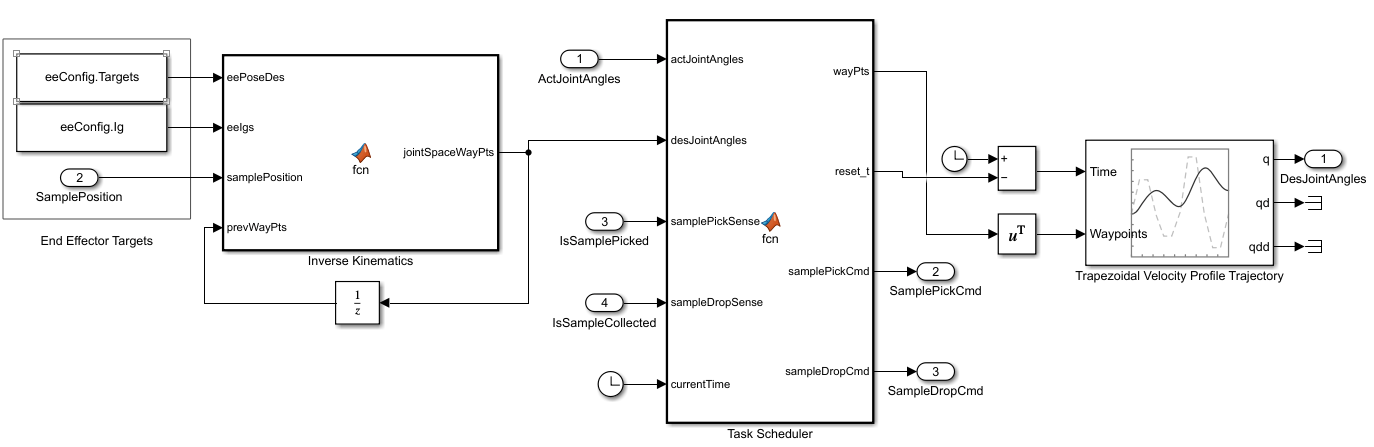#### Manipulator Simulation Results

Results showing the end effector trajectory passing through the desired waypoints and the comparison between desired and actual actuator joint angles. More quantities can be viewed at Manipulator Sensing subsystem in the model.### References

 Filip, Jan, Martin Azkarate, and Gianfranco Visentin. "Trajectory control for autonomous planetary rovers." In 14th Symposium on Advanced Space Technologies in Robotics and Automation (ASTRA). 2017.

 Snider, Jarrod M. "Automatic steering methods for autonomous automobile path tracking." Robotics Institute, Pittsburgh, PA, Tech. Rep. CMU-RITR-09-08 (2009).

 X. Wu, L. Yang and M. Xu, "Speed following control for differential steering of 4WID electric vehicle," IECON 2014 - 40th Annual Conference of the IEEE Industrial Electronics Society, 2014, pp. 3054-3059, doi: 10.1109/IECON.2014.7048945.